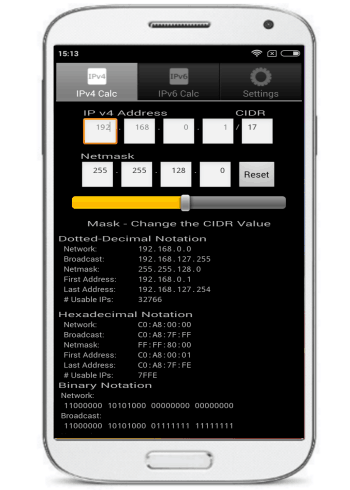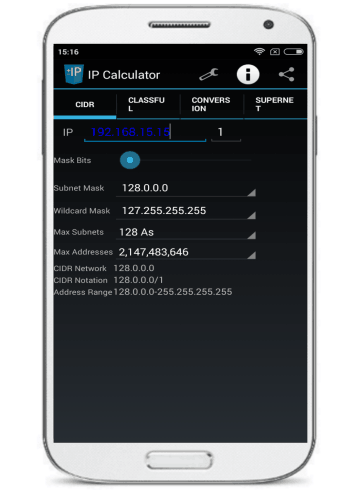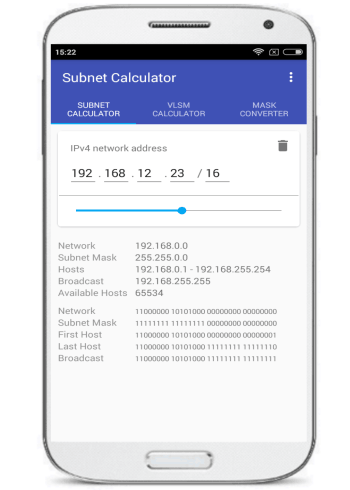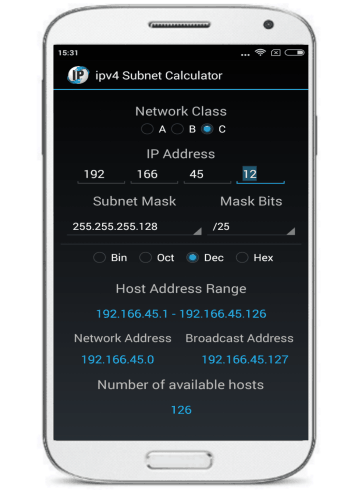Editor Ratings:
User Ratings:
[Total: 1 Average: 5]

Here is a list of 5 free Android IP subnet calculator apps. You can use these IP subnet calculators for Android to calculate subnets info, like, number of maximum hosts in a subnet, subnet address, subnet broadcast address, a range of IP addresses that can be allocated to each host in a subnet and many other parameters. All of the subnet calculators included in this list are very easy to use and each of these calculator updates the result in the real time as per your inputs. Most of these also allow you to share the calculated subnet results or copy them to the clipboard. I have also included some advanced IP subnet calculators that also allow you to calculate VLSM, convert IP address to different notations, Mask converter, etc and some also give you the option to view the results in different numerical systems like decimal, dotted-decimal, hexadecimal, binary, etc.If you are looking for IP subnet calculator for Windows, then do not forget to check out these 5 free IP subnet calculator for Windows. These IP subnet calculators can be easily used by any professional or students to calculate subnets.

Let’s now see these 5 free Android IP subnet calculator apps:

## ipv4 Subnet Calculator:ipv4 Subnet Calculator is a free Android IP subnet calculator to calculate subnets. This free Android IP subnet calculator will calculate a range of addresses that you can assign to each of the hosts in subnetworks. It will also display the network address, broadcast address, and the complete range of addresses of each subnet in the network. You can copy the results to the clipboard and then export it to external applications.

Getting started with this Android IP subnet calculator is really simple. After installing, specify an IP address and it will then automatically calculate all the subnets. After that, you can adjust other parameters like Subnet mask and CIDR and it will then automatically calculate and update the results. It displays the results in 2 formats i.e. Decimal and Binary. You can view the address range of each subnet from the Networks tab in the results section. You can long press on the results section to copy the results to the clipboard.

## IP Network Calculator:The best part is that it gives you the option to share the calculation results with others. To share or copy the results, long press on the results section and then choose an option from the menu to share all results from all formats, or just a particular parameter like Network address or Netmask Address.

## Network IP/Subnet Calculator:Network IP/Subnet Calculator is a more advanced subnet calculator as compared to others included in my list. Apart from calculating subnets, you can also use it to convert IP address from one notation to another, supernets, and classful subnetting. It comes with a nice and clean interface as compared to other subnet calculators included in my list.

It is very easy to use this app. Switch to the CIDR tab in the app and specify an IP address and the number of mask bits. It will then automatically update the other parameter fields like Subnet mask, Wildcard mask, Max subnets, and a maximum number of addresses. It also displays CIDR (Classless Inter-Domain Routing) Network address, Notation and Address range. It auto updates the results in real-time as you adjust the other parameters in the calculator.

## VLSM/CIDR Subnet Calculator:VLSM/CIDR Calculator is another IP Subnet Calculator app for Android. You can also use it to calculate VLSM (Variable Length Subnet Mask) and convert Subnet mask to Wildcard Mask. Use its SUBNET CALCULATOR tab and then specify an IP address and mask bits. It will then automatically display the subnet calculation results. From its result section, you can see the Network address, Subnet Mask, an address range of the hosts in the subnet, and the broadcast address of the subnetwork. It also displays the results in binary format. Unfortunately, it doesn’t give you the option to share the results or copy the results to the clipboard.

## ipv4 Subnet Calculator:ipv4 Subnet Calculator is another simple IP subnet calculator app for Android. Like other Android IP subnet calculator apps included in my list, it will also display subnet network address, broadcast address, total number of available hosts, and the complete host address range. You can view the results in 4 different number system i.e. Dec (Decimal), Bin (Binary), Hex (Hexadecimal), and Oct (Octal). You can also copy the subnet calculation results to the clipboard.

Getting started with this free Android app is really easy, download it from the link provided above and install it. After that, simply specify the IP address and mask bits and it will then automatically display the calculated subnetwork details like address range, network address, available hosts, etc. To view the results in the different number system, select a number system below the Subnet Mask fields and it will then automatically convert the results to the selected number system.

## Verdict:

In this article, I have introduced you to 5 free Android IP subnet calculator apps. Personally, I liked ipv4 Subnet Calculator and IP Network Calculator. ipv4 Subnet Calculator also displays the IP address range of each of the subnets that we can create and IP Network Calculator allows you to share the entire calculated results. If you need an advanced IP subnet calculator then I will recommend you to either go with VLSM/CIDR Calculator or Network IP/Subnet Calculator.

 Editor Ratings: User Ratings:[Total: 1 Average: 5]